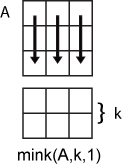Documentation

mink

Find k smallest elements of array

Description

example

B = mink(A,k) returns the k smallest elements of A.

• If A is a vector, then mink returns a vector containing the k smallest elements of A.

• If A is a matrix, then mink returns a matrix whose columns contain the k smallest elements of each column of A.

• If A is a multidimensional array, then mink returns the k smallest elements along the first dimension whose size does not equal 1.

example

B = mink(A,k,dim) determines the k smallest elements of A along dimension dim.

example

B = mink(___,'ComparisonMethod',c) optionally specifies how to compare elements of A for any of the previous syntaxes. For example, mink(A,k,'ComparisonMethod','abs') returns the k smallest elements of A according to their absolute values.

example

[B,I] = mink(___) finds the indices of the smallest k values of A and returns them in I.

Examples

collapse all

Compute the smallest 3 elements of a vector.

A = 1:10;
B = mink(A,3)
B = 1×3

1     2     3

Compute the smallest 3 elements of each row of a matrix.

A = magic(5)
A = 5×5

17    24     1     8    15
23     5     7    14    16
4     6    13    20    22
10    12    19    21     3
11    18    25     2     9

B = mink(A,3,2)
B = 5×3

1     8    15
5     7    14
4     6    13
3    10    12
2     9    11

Compute the 2 smallest elements of a complex vector according to their magnitude, and return the indices where they are located in the input vector.

A = [2-2i 5+i -7-3i -1+i]
A = 1×4 complex

2.0000 - 2.0000i   5.0000 + 1.0000i  -7.0000 - 3.0000i  -1.0000 + 1.0000i

[B,I] = mink(A,2,'ComparisonMethod','abs')
B = 1×2 complex

-1.0000 + 1.0000i   2.0000 - 2.0000i

I = 1×2

4     1

Input Arguments

collapse all

Input array, specified as a vector, matrix, or multidimensional array.

• If A is a vector, then mink returns a vector containing the k smallest elements of A.

• If A is a matrix, then mink returns a matrix whose columns contain the k smallest elements of each column of A.

• If A is a multidimensional array, then mink returns the k smallest elements along the first dimension whose size does not equal 1.

If A has type categorical, then it must be ordinal.

Data Types: single | double | int8 | int16 | int32 | int64 | uint8 | uint16 | uint32 | uint64 | logical | char | categorical | datetime | duration
Complex Number Support: Yes

Number of minima to return, specified as a positive integer scalar. If k is greater than or equal to the number of elements in the operating dimension, then mink sorts the input array along that dimension.

Data Types: single | double | int8 | int16 | int32 | int64 | uint8 | uint16 | uint32 | uint64

Operating dimension, specified as a positive integer scalar. By default, mink operates along the first dimension whose size does not equal 1.

For example, if A is a matrix, then mink(A,k,1) operates along the rows of A, computing minimums for each column.mink(A,k,2) operates along the columns of A, computing minimums for each row.Data Types: single | double | int8 | int16 | int32 | int64 | uint8 | uint16 | uint32 | uint64

Comparison method, specified as one of the following:

• 'auto' — Compare elements of input A by real(A) when A is real, and by abs(A) when A is complex.

• 'real' — Compare elements of input A by real(A) when A is real or complex. If A has elements with equal real parts, then use imag(A) to break ties.

• 'abs' — Compare elements of input A by abs(A) when A is real or complex. If A has elements with equal magnitude, then use angle(A) in the interval (-π,π] to break ties.

Output Arguments

collapse all

Output array, returned as a scalar, vector, matrix, or multidimensional array. mink returns the k elements in order from smallest to largest.

Index array, returned as a vector, matrix, or multidimensional array. I is the same size as B. If the output array B contains repeated elements, then the order of their indices in I matches the order in which they appear in the input array.